﻿ Increment Primes

### Increment Primes

P.M. MazurkinOPEN ACCESSPEER-REVIEWED

## Increment Primes

Doctor of Engineering Science, Academician of RANS, member of EANS, Volga Region State Technological University, Russia

### Abstract

The increment of prime numbers was a clear indication. Increase - the number increases, the addition of something. If the number of prime numbers, figuratively called the ladder of Gauss-Riemann, the increase may well be likened to the steps, separated from the ladder itself. We prove that the law is obeyed z2(i2=2)=1/2-1/2cos(πP(n)/2) in the critical line i2=2 of the second digit binary number system. This functional model was stable and in other quantities of prime numbers (3000 and 100 000). The critical line is the Riemann column i2=2 binary matrix of a prime rate. Not all non-trivial zeros lie on it. There is also a line of frames, the initial rate (yields patterns of symmetry) and left the envelope binary number 1. Cryptographers cannot worry: even on the critical line growth of prime numbers z2i=1/2-1/2cos(πPj/2) contain the irrational number π=3.14159….

### At a glance: Figures

1234
Prev Next

• Mazurkin, P.M.. "Increment Primes." American Journal of Applied Mathematics and Statistics 2.2 (2014): 66-72.
• Mazurkin, P. (2014). Increment Primes. American Journal of Applied Mathematics and Statistics, 2(2), 66-72.
• Mazurkin, P.M.. "Increment Primes." American Journal of Applied Mathematics and Statistics 2, no. 2 (2014): 66-72.

 Import into BibTeX Import into EndNote Import into RefMan Import into RefWorks

### 1. Introduction

Gauss, Riemann, and behind them and other mathematicians carried away by the relative power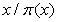of prime numbers with a truncated start, represented in dotted decimal notation. In this case, apparently unconsciously, this figure has been expressed with the logarithm of the irrational basis, and thus the transition from ten degrees to its natural logarithm of false identification has occurred. It is the main error of more than 150 years.

Applicationand false idea that future discharges of the decimal system the number of primes all the time increases to about 2.3, based on the assumption that~. And the reason for this turn in the study of prime numbers has been rather prosaic. As noted in : "Gauss, the greatest of mathematicians discovered the law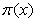~the age of fifteen, studying tables of primes contained in the gift to him a year before the table of logarithms."

We refused to logarithms, went to the binary system. It turned out that the very prime,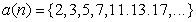,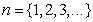is not sufficiently effective measure. To avoid any claims to the proof, we adopt this traditional range.

### 2. The Increment of Prime Numbers

This new figure was visible and at the same time is mathematically equivalent to a series of prime numbers. Increment - the number increases, the addition of something. If the number of primeslong figuratively called the "ladder of Gauss-Riemann", the increase may well be likened to the steps, separated from the carrier farm the base of the stairs. A long and tall ladder physically may well contain two parts - apart from the construction of stairs and a separate farm grounds.

### 3. Algorithm Building a Number of Prime Numbers

He is widely known, has the form(1)

where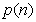- the increment of a prime number,- the order of (number) of a prime number. The very number of primes is given initially, it is determined by the condition of the indivisibility of the other numbers, except on unit and itself (the latter condition, even excessive).

Therefore, growth is always calculated by subtracting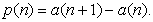(2)

### 4. 500 Prime Numbers

In Table 1 shows fragments of the increment of a number of. Among the 500 prime numbers was a maximum increasefor a prime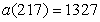with code 100010 in binary.

The fundamental difference of a number of increment of the number of primes is that in the increment (the same number - an abstract measure of the amount), only one column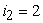bit binary numbers is completely filled and critical, and the first class has only zeros for the set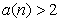. Full filling will continue to infinity, therefore, can be considered a proven fact the appearance of the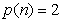at any power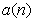.

### 5. Mathematical Landscape

To construct (Figure 1) we take the exampleand delete those rows in which the five columns contains at least one trivial zero.

An indicator is a binary number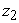in the field of real numbers (0, 1).

### 6. Critical Line

The first line in Table 1 will automatically fall out of the set. After that, at any length series of prime numbers the first columnis zero. Then each value increment from right to left starting from zero and ends with the unit. And for the unit as a wave broken lines are only trivial zeros. All non-trivial zeros are arranged in any row between 1 (left) and 0 (first column on the right). Then Riemann's critical line in a vertical column. But it is clear that not all non-trivial zeros lie on the critical line. They are available in other binary digits, interspersed with trivial zeros.

Figure 1. The landscape of increment in the number of 500 prime numbers

### 7. Critical Start of the Series

In Table 2 shows the three critical primes.

#### Table 2. Gain critical primes at the beginning of a series

Together with Table 1 critical prime numbers give a full range of prime numbers, which this article is not considered. To accept it, you must: a) to recognize as simple that number which shares only on unit (zero/zero indefinite); b) change the order in a number; c) gain 1 is a border in the uncritical range includes non-critical prime.

Further detailed analysis of the increment will fulfill a number of non-critical primes.

### 8. Effect of Discharge i2

In the software environment of Excel sum over the columns in Table 1 (excluding the first line) and get the number of units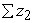in the ranks of the binary system.

Model should give the relative values that allow comparison between different series of increment of prime numbers. After the identification of bio-law  was to teach the following conclusions:

- the share of units (Figure 2) lines of the binary matrix of increment of prime numbers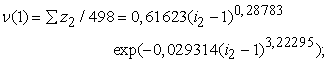(3)

- the proportion of zeros in (Figure 2) lines of the binary matrix of increment of prime numbers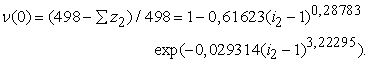(4)

#### Table 3. Influence of discharge binary system (498 lines)

In favor of computing the number of units, there are two distinctive features:

1) the number of zeros (trivial and nontrivial) is almost three times as many units (Table 3);

2) the design of the formula (3) is easier compared with the expression (4).

Apparently, the option is 0,61623 with increasing number ofwill approach to the golden ratio 0,618 .... Then, on the critical line are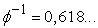ones and 0,6182 zeros.

Contribution amounts for units of columns (Figure 3) to the total (Table 3, 773) will be equal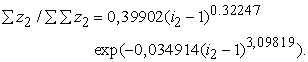(5)

On the critical linecontribution approaching to the square of the golden section.

### 9. Influence of Increment

The explanatory variable we take the increase of a prime number. Then on the different digits of the binary number system formed their statistical model (Table 4) type(6)

where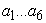- the parameters of the model (6).

If we ignore the first and last bits binary system, the closest to a rational number 1/2 on real values is the discharge. For the critical lineequation (6) is reduced (Figure 4) to the form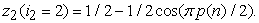(7)

Thus completes the proof of the Riemann hypothesis and remove the message from the Internet: "Here the famous Riemann hypothesis, that the real part of the root is always exactly equal to 1/2, no one has yet proven, although the proof of it would have been for the theory of prime numbers in the highest degree the importance. At the present time, the hypothesis is verified for seven million of the roots".

With increasing power of prime numbers equation (7) for the critical line continues, but the graphs such as Figure 4 will be more frequent fluctuations due to higher growth. The increment is growing much more slowly than simple numbers. This will increase the power of the series.

### 10. The Binary Number for Non-emergency Lines

Table 4 shows the parameters of equation (6).

To model the formula (6) patternsof Table 1 excludes those lines that are in the column trivial zeros (empty cells). Then there is an array of ones and non-trivial zeros. With the increase in the discharge of a binary number of lines in the arraywill be reduced. Graphs are shown in Figure 5.

Zeros and ones are grouped together. Because of the small number of primes in an array 500 in column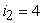Table 1, as seen from the right graph in Figure 5, formed only two complete groups of four elements. Therefore formula (5) gets a full design.

To discharge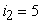the number of groups of ones and zeros (Figure 6) is clearly insufficient.

At the top there was formed the group of seven units, but at the bottom of the group of zeros is only being formed. Therefore we can define a rational power series of prime numbers, providing all the bits. The data in Table 4 shows that forthe required 710 prime numbers (more than 708,9489).

It is noticed that while reducing the data set atto 36 lines of the character of the formulas in columnsandis not changed. This indicates the saturation of these bits are the number of groups of ones and zeros. They are sufficient to identify patterns (6) with parameters from Table 4.

Then the third category with an increase in powergets the physical meaning of the(8)

as shear waves 0,78539815 almost coincides with the value of the angle of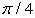= 0,7853975….

### 11. Check the Law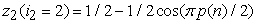. On the critical lineindicated this model is stable and the other quantities of prime numbers (Figure 7).

With the increase in power series to 3000 increases the number of points in the graph. To test the subset was taken (1704 lines) increments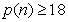from 100 000 prime numbers. It follows that in any sample observed our law (6) of the critical line.

### 12. The Minimum Sample of Prime Numbers

The method of cutting off the bottom of Table 1 (Table 5) determine the minimum sample, where the law still in effect sustained the critical line.

#### Table 5. The minimum number of prime numbers

The minimum number of non-critical primes form only three members, which was obtained by equation (5) with rational parameters given in Table 6.

Approximation error of 0.51/2 is negligible. Schedule a simple in construction of equation (6) is shown in Figure 8.

In other dischargesshould be increasing the number of (power) of primes.

Between increment and its component there is a pattern of transition of the numbers from the decimal system of notation to binary.

### 13. Benchmarks

The first unit of the left formed the asymptotic line to the left of which there are only trivial zeros. Consider the benchmarks in the 500 prime numbers.

Benchmarks form a block. In an array of 500 points are few (Table 7), only five.

Proceeding from the condition that in the beginning of the series the gain is equal to the unit, was obtained (Figure 9) the formula(9)

where the index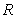denotes a fixed points a prime number.

The use of benchmarks is much more compact than a relationship.

### 14. Primary Increment

This - the third parameter (the first - a critical line 1/2), giving a picture of the increment rate of prime numbers. Parameter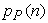for a number of 100 000 prime numbers are shown in Table 8, and he compiled the first appearance of the subsequent term. Primary increment is irregular, for example, an increase of 14 comes after 8 and earlier values of 10 and 12.

Various font allocated triangles (patterns of geometry) with sides (with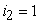- non-trivial zeros). Then the harmonious geometrical structures define the algorithm capacity increment, and even prime number.

Line increment varies with the initial constant "deuce", and there will be fluctuations, the trend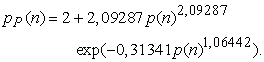(10)

For conditions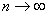will always be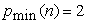.

### 15. The Envelope of the Line

Increments to the left of the asymptotic lines have trivial zeros. Therefore, taken into account the wave envelope line, which in different places concerns a critical line.

This - the fourth parameter of the series.

Divide the increase in two parts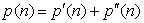. On the envelope line by line in the table (Figure 8) are located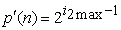. And in the blocks.

The trend with unit from the formula with three fluctuations looks like(11)

#### Table 8. The primary increase in the number of 100 000

Atin the formula (11) always will be in the beginning 1.

### 16. Conclusions

The critical line Riemann is located in a vertical columnbinary matrix of increment of number of simple. Not all non-trivial zeros lie on it. There is also a line of benchmarks, the initial rate (giving patterns of symmetry) and the bending around.

### References

  Don Zagier. The first 50 million prime numbers. URL: http://www.ega-math.narod.ru/Liv/Zagier.htm.In article  Mazurkin P.M. Biotechnical principle and stable distribution laws // Successes of modern natural sciences. 2009. № 9, 93-97.In article